# mne.bem.ConductorModel#

class mne.bem.ConductorModel[source]#

BEM or sphere model.

Attributes:
`radius`

Sphere radius if an EEG sphere model.

Methods

 `__contains__`(key, /) True if the dictionary has the specified key, else False. `__getitem__` x.__getitem__(y) <==> x[y] Implement iter(self). Return len(self). Return copy of ConductorModel instance. `fromkeys`(iterable[, value]) Create a new dictionary with keys from iterable and values set to value. `get`(key[, default]) Return the value for key if key is in the dictionary, else default. `pop`(key[, default]) If the key is not found, return the default if given; otherwise, raise a KeyError. Remove and return a (key, value) pair as a 2-tuple. `setdefault`(key[, default]) Insert key with a value of default if key is not in the dictionary. `update`([E, ]**F) If E is present and has a .keys() method, then does: for k in E: D[k] = E[k] If E is present and lacks a .keys() method, then does: for k, v in E: D[k] = v In either case, this is followed by: for k in F: D[k] = F[k]
__contains__(key, /)#

True if the dictionary has the specified key, else False.

__getitem__()#

x.__getitem__(y) <==> x[y]

__iter__(/)#

Implement iter(self).

__len__(/)#

Return len(self).

clear() None.  Remove all items from D.#
copy()[source]#

Return copy of ConductorModel instance.

fromkeys(iterable, value=None, /)#

Create a new dictionary with keys from iterable and values set to value.

get(key, default=None, /)#

Return the value for key if key is in the dictionary, else default.

items() a set-like object providing a view on D's items#
keys() a set-like object providing a view on D's keys#
pop(key, default=<unrepresentable>, /)#

If the key is not found, return the default if given; otherwise, raise a KeyError.

popitem(/)#

Remove and return a (key, value) pair as a 2-tuple.

Pairs are returned in LIFO (last-in, first-out) order. Raises KeyError if the dict is empty.

Sphere radius if an EEG sphere model.

setdefault(key, default=None, /)#

Insert key with a value of default if key is not in the dictionary.

Return the value for key if key is in the dictionary, else default.

update([E, ]**F) None.  Update D from dict/iterable E and F.#

If E is present and has a .keys() method, then does: for k in E: D[k] = E[k] If E is present and lacks a .keys() method, then does: for k, v in E: D[k] = v In either case, this is followed by: for k in F: D[k] = F[k]

values() an object providing a view on D's values#

## Examples using `mne.bem.ConductorModel`#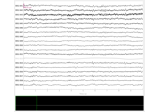Setting the EEG reference

Setting the EEG reference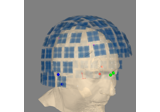Source alignment and coordinate frames

Source alignment and coordinate frames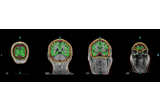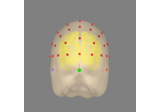EEG forward operator with a template MRI

EEG forward operator with a template MRI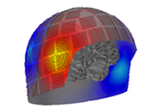Brainstorm Elekta phantom dataset tutorial

Brainstorm Elekta phantom dataset tutorial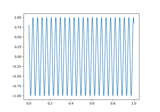Brainstorm CTF phantom dataset tutorial

Brainstorm CTF phantom dataset tutorial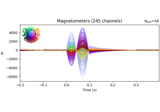4D Neuroimaging/BTi phantom dataset tutorial

4D Neuroimaging/BTi phantom dataset tutorial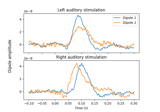Computing source timecourses with an XFit-like multi-dipole model

Computing source timecourses with an XFit-like multi-dipole model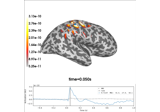Optically pumped magnetometer (OPM) data

Optically pumped magnetometer (OPM) data